# Solution assignment 09 Quadratic inequalities

### Assignment 9

For which value(s) ofdo the parabola: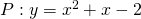and the line: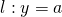have no points in common?

### Solution

First we calculate the intersection points of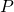and. Thus we have to solve: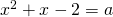This equation may not have common points so the discriminant of quadratic equation has to be less than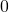: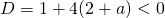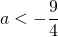For these values ofthe parabolaand the linehave no common points.